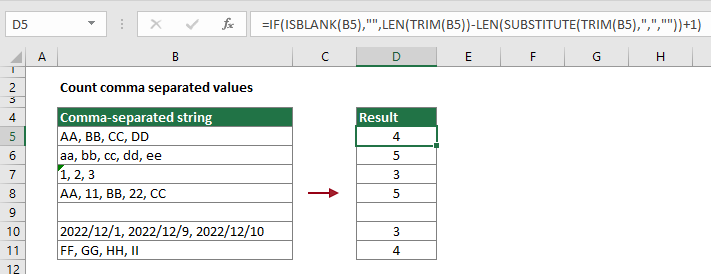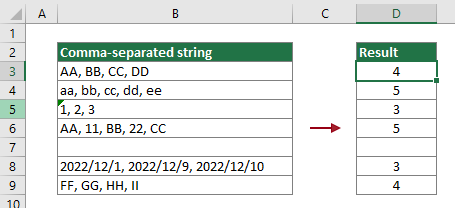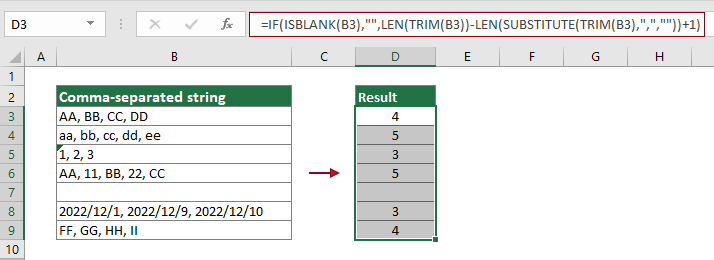Note: The other languages of the website are Google-translated. Back to English

## Count comma separated values in a cell

In this tutorial, you will learn how to use a combination of the LEN, TRIM and SUBSTITUTE functions to count comma separated values in a cell in Excel.#### How to count comma separated values in a cell in Excel?

As the below screenshot shown, there is a comma-separated string list in column B which you want to count the comma separated values in each cell, please do as follows to achieve it.Generic formula

=IF(ISBLANK(B3),"",LEN(TRIM(B3))-LEN(SUBSTITUTE(TRIM(B3),",",""))+1)

Arguments

A1: Represents the cell containing the comma-separated string which you will count the comma separated values.

How to use this formula?

1. Select a blank cell to output the result. In this case, I select cell D3.

2. Enter the below formula into it and press the Enter key. And then drag its Fill Handle all the way down to apply it to other cells.

=IF(ISBLANK(B3),"",LEN(TRIM(B3))-LEN(SUBSTITUTE(TRIM(B3),",",""))+1)Note: In this formula, B3 is the cell containing the comma-separated string you will count comma separated values inside. You can change it as you need.

How does this formula work?

=IF(ISBLANK(B3),"",LEN(TRIM(B3))-LEN(SUBSTITUTE(TRIM(B3),",",""))+1)

1. LEN(TRIM(B3)): The TRIM function removes all extract spaces from the comma-separated string "AA, BB, CC, DD", and only keeps single space between words. And then the LEN function calculates the total length of "AA, BB, CC, DD" and returns the result as 14;

2. LEN(SUBSTITUTE(TRIM(B3),",",""))

• SUBSTITUTE(TRIM(B3),",",""): As the above explanation shown, the TRIM function here removes all extract space from "AA, BB, CC, DD" and returns the result as "AA, BB, CC, DD". And then the SUBSTITUTE function replaces all commas in "AA, BB, CC, DD" with nothing and get the result "AA BB CC DD";
• LEN("AA BB CC DD"): The LEN function returns the number of characters in a text string "AA BB CC DD". The result is 11.

3. 14-11+1: Here use the total length of "AA, BB, CC, DD" subtracting the total length of "AA BB CC DD" to get the total number of commas in the string (14-11=3). As there always be a word after the last comma, you need to add 1 to number 3 to get the total comma separated words in the string. Here the result is 4.

4. IF(ISBLANK(B3),"",14): The If function here says if B3 is empty, then return nothing, otherwise return the number 14. Here B3 contains values, so the IF function returns the number 14.

#### Related functions

Excel LEN function
The Excel LEN function returns the number of characters in a text string.

Excel SUBSTITUTE function
The Excel SUBSTITUTE function replaces text or characters within a text string with another text or characters.

Excel TRIM function
The Excel TRIM function removes all extra spaces from text string and only keeps single spaces between words.

#### Related formulas

Count occurrences of specific characters in an Excel cell
This tutorial introduces how to apply formulas based on the LEN and SUSTITUTE functions to count the number of times a specific character appears in an Excel cell.

Count specific characters in a range of cells
This tutorial introduces some formulas to count the number of times a specific character appears in a range in Excel.

Count specific words in a cell in Excel

Count specific words in a range in Excel
This article explains a formula to count the number of times a specific word appears in a range of cells in Excel.

Count number of characters in a cell in Excel
The total characters not only includes all letters, but also all spaces, punctuation marks and symbols in the cell. Follow this tutorial to easily count the total characters in a cell with the LEN function in Excel.

Count number of characters in a range in Excel
This article explains a formula to count total characters that appear in a range of cells in Excel.

### The Best Office Productivity Tools

#### Kutools for Excel - Helps You To Stand Out From Crowd

Would you like to complete your daily work quickly and perfectly? Kutools for Excel brings 300 powerful advanced features (Combine workbooks, sum by color, split cell contents, convert date, and so on...) and save 80% time for you.

• Designed for 1500 work scenarios, helps you solve 80% Excel problems.
• Reduce thousands of keyboard and mouse clicks every day, relieve your tired eyes and hands.
• Become an Excel expert in 3 minutes. No longer need to remember any painful formulas and VBA codes.
• 30-day unlimited free trial. 60-day money back guarantee. Free upgrade and support for 2 years.#### Office Tab - Enable Tabbed Reading and Editing in Microsoft Office (include Excel)

• One second to switch between dozens of open documents!
• Reduce hundreds of mouse clicks for you every day, say goodbye to mouse hand.
• Increases your productivity by 50% when viewing and editing multiple documents.
• Brings Efficient Tabs to Office (include Excel), Just Like Chrome, Firefox, And New Internet Explorer.